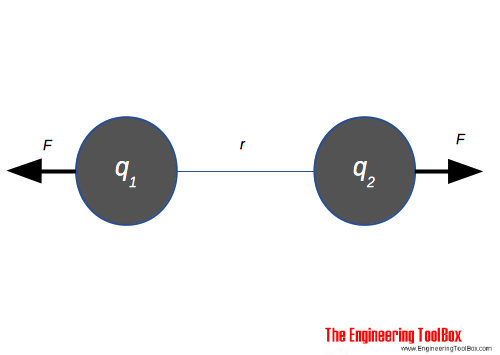Engineering ToolBox - Resources, Tools and Basic Information for Engineering and Design of Technical Applications!

# Coulomb's Law

## The electric force acting on a point charge.Coulomb's law calculates the electric force acting between to electric charges  q1 and q2 and can be expressed as

F = k q1 q2 / r2

= q1 q2 / (4 π ε0 r2)    (1)

where

F = force of attraction or repulsion (N)

k = Coulomb's constant = 1 / (4 π ε0) = 8.9875517873681764 × 109 (Nm2/C2) in air

q = charge (Coulombs, C)

r = distance between charges (m)

The quantity of charge (number of electrons) is measured in the unit Coulomb - C - where

1 coulomb (C) = 6.24 1018 electrons

The smallest charge that exists is the charge carried by an electron equal to -1.602 10-19 coulomb.

### Example - Force between two Charged Bodies

Two spheres in air with distance 20 mm is both charged with 2 μC (2 10-6 C). The force acting between the two spheres can be calculated as

F = (8.98755 109 Nm2/C2) ((2 μC) (10-6 C/μC)) ((2 μC) (10-6 C/μC)) / ((20 mm)(10-3 m/mm))2

= 90 N

## Related Topics

• Electrical - Electrical units, amps and electrical wiring, wire gauge and AWG, electrical formulas and motors.

## Engineering ToolBox - SketchUp Extension - Online 3D modeling!

Add standard and customized parametric components - like flange beams, lumbers, piping, stairs and more - to your Sketchup model with the Engineering ToolBox - SketchUp Extension - enabled for use with the amazing, fun and free SketchUp Make and SketchUp Pro .Add the Engineering ToolBox extension to your SketchUp from the SketchUp Pro Sketchup Extension Warehouse!

Translate

## Privacy

We don't collect information from our users. Only emails and answers are saved in our archive. Cookies are only used in the browser to improve user experience.

Some of our calculators and applications let you save application data to your local computer. These applications will - due to browser restrictions - send data between your browser and our server. We don't save this data.

## Citation

• Engineering ToolBox, (2013). Coulomb's Law. [online] Available at: https://www.engineeringtoolbox.com/coulomb-law-electric-charge-force-d_1855.html [Accessed Day Mo. Year].

Modify access date.

. .

#### Scientific Online Calculator3 30